# Functional equation of an $L$-function

The Riemann $\zeta$-function has functional equation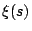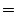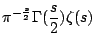(1)

Dirichlet $L$-functions satisfy the functional equation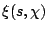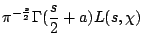(2)

where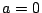if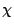is even and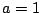ifis odd, and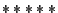.

The Dedekind zeta function of a number field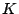satisfies the functional equation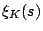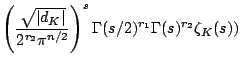(3)

Here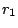and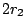are the number of real and complex conjugate embeddings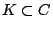,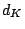is the discriminant, and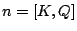is the degree of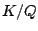.

$L$-functions associated with a newform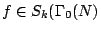satisfy the functional equation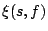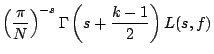(4)

whereifis even andifis odd.

$L$-functions associated with a Maass newform with eigenvalue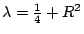on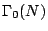satisfy the functional equation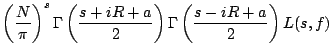(5)

whereif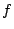is even andifis odd.

$GL(r)$ $L$-functions satisfy functional equations of the form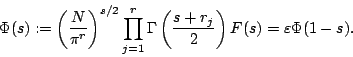[This section needs a bit of work]

Back to the main index for The Riemann Hypothesis.